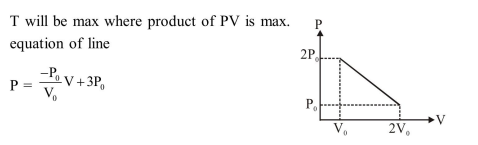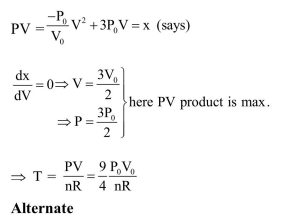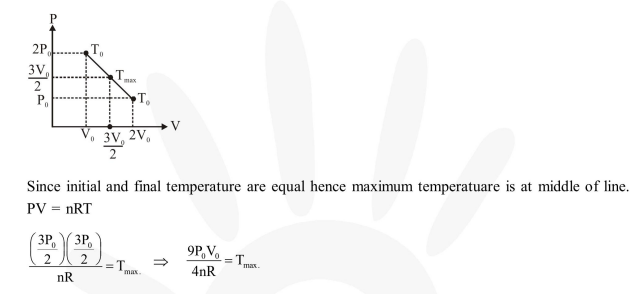Deepak Scored 45->99%ile with Bounce Back Crack Course. You can do it too!

# Solve this following

Question:

' $n$ ' moles of an ideal gas undergoes a process $\mathrm{A} \rightarrow \mathrm{B}$ as shown in the figure. The maximum temperature

of the gas during the process will be :

1. $\frac{9 \mathrm{P}_{0} \mathrm{~V}_{0}}{\mathrm{nR}}$

2. $\frac{9 \mathrm{P}_{0} \mathrm{~V}_{0}}{4 \mathrm{nR}}$

3. $\frac{3 \mathrm{P}_{0} \mathrm{~V}_{0}}{2 \mathrm{nR}}$

4. $\frac{9 \mathrm{P}_{0} \mathrm{~V}_{0}}{2 \mathrm{n} \mathrm{R}}$

Correct Option: , 2

Solution: